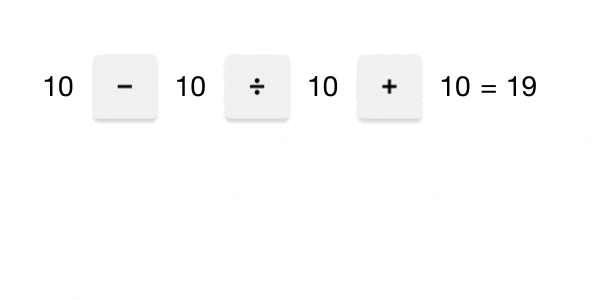Back

## Even to Odd

What if we had an equation where the numbers on one side of the equation didn't change but the arithmetic operations among them did? As the operations changed, the result on the other side would change, too.To solve an operator search puzzle, you need to find the operations that make the equation have a desired result. Keep reading to see a few examples with strategies used to solve them, or jump ahead to today's challenge if you feel ready.

Let's start by looking at a puzzle where only addition and subtraction are allowed. Is it possible to replace every square with either a $+$ or a $-$ to make the equation true:

$2\ \square\ 3\ \square\ 5\ \square\ 6 = 10?$

Let's develop some reasoning we can extend to more complex puzzles. The maximum value we can get is $2 + 3 + 5 + 6 = 16,$ which is too large by six. What if we subtract six instead of adding it? That would give us $2 + 3 + 5 - 6 = 4,$ which is too small... again, by six. Changing the sign immediately before the $6$ decreased the result by $2 \times 6 = 12.$

Let's change it back to $2 + 3 + 5 + 6 = 16,$ and note that we want to decrease the result by $16 - 10 = 6.$ Changing the $+$ sign in front of the $3$ should do it, since $2 \times 3 = 6.$ Let's try it:

$2 - 3 + 5 + 6 = 10.$

This indeed works. Feel free to play with an interactive version of the same puzzle below, clicking on the operators to change them. Does the parity of the result ever change?

With more operations, things get more complicated. If we replace each square with a $+,\ -,\ \times$ or a $\div,$ can we make this equation true:

$8\ \square\ 4\ \square\ 2\ \square\ 12\ \square\ 4 = 3?$

Let's think about the parities of the numbers. The result is odd, while every other number is even. The only way we can get an odd number from two even numbers is by dividing them, so at least one of the squares must be replaced with a $\div$ symbol. The only pair of consecutive numbers with an odd quotient are $12$ and $4,$ so let's put a $\div$ symbol between them. Multiplication and division are performed before addition and subtraction according to the order of operations, so we can go ahead and simplify $12 \div 4:$

\begin{aligned} 8\ \square\ 4\ \square\ 2\ \square\ 12 \div 4 & = 3 \\ 8\ \square\ 4\ \square\ 2\ \square\ 3 & = 3. \end{aligned}

Our puzzle looks a little easier now that we've simplified it. We know that $8 = 4 \times 2,$ so $8 - 4 \times 2 = 0:$

\begin{aligned} 8 - 4 \times 2\ \square\ 3 & = 3 \\ 0\ \square\ 3 & = 3 \\ 0 + 3 & = 3. \end{aligned}

We can then work backwards and substitute symbols for the original equation's squares to make it balance:

$8 - 4 \times 2 + 12 \div 4 = 3.$

Below is an interactive version of the puzzle.

Finally, let's look at a puzzle allowing a new operation: concatenation. When we concatenate a pair of numbers, we slide them together. For example, $2 || 3 = 23$ and $12 || 80 = 1280.$ Can we make the equation true if we're limited to $+, -,$ and $||$ operators:

$2\ \square\ 2\ \square\ 2\ \square\ 2 = 44?$

There's no way we can get a result as large as $44$ using only addition and subtraction, so we need to use at least one $||$ symbol somewhere. While $2 || 2 = 22$ is closer, it's still too small. Meanwhile, $2 || 2 || 2 = 222$ is far too large, so we can't have two or more $||$ symbols in a row. Noticing that $2 || 2 = 22$ is half of $44,$ we can add two copies of $2 || 2:$

$2\ ||\ 2 + 2\ ||\ 2 = 44.$

Below is an interactive version of this puzzle.

Now, try your hand at today's operator search challenge.

# Today's Challenge

In the equation below, click on the operators to change them to any combination of $+, -, \times, \div$ and ||.

Your goal is to make an odd, negative number with the largest possible absolute value. How low can you go?

The order of operations for these five operators is concatenation, then multiplication and division, then addition and subtraction.×